• 语言自动机考试卷，包括2018年的半期考试考试卷与一些期末试卷
• 北邮形式语言自动机考试卷，包括2018年的半期考试考试卷与一些期末试卷
• 形式语言自动机——图灵机一PPT学习教案.pptx学习课件
• 形式语言自动机课件，通俗易懂，容易掌握，轻松得高分
• 形式语言自动机——上下文无关文法与下推自动机四PPT学习教案.pptx学习课件
• 形式语言自动机课程笔记 学到编译原理的时候用到了文法相关概念，复习自动机正好把以前的笔记整理一下也贴上来 一、集合及集合的基本运算 （0）集合 集合（set）：一组可区分的对象的全体称为集合，而这些对象...
形式语言自动机课程笔记学到编译原理的时候用到了文法相关概念，复习自动机正好把以前的笔记整理一下也贴上来

文章目录
一、集合及集合的基本运算（0）集合（1）交并差补（2）幂集（3）笛卡尔积（4）关系
二、字母表，字符串和语言（1）字母表（2）字符串（3）语言

一、集合及集合的基本运算
（0）集合
集合（set）：一组可区分的对象的全体称为集合，而这些对象称为集合的元素（element）集合的描述：
描述方式适用规则例子列举法元素个数较少的集合直接把元素全部列出S={a,b,c,d}形式化描述元素个数较多的集合特别是由无穷多个元素组成的集合{x│P(x)}S={n│n是偶数}
（1）交并差补
一张图不解释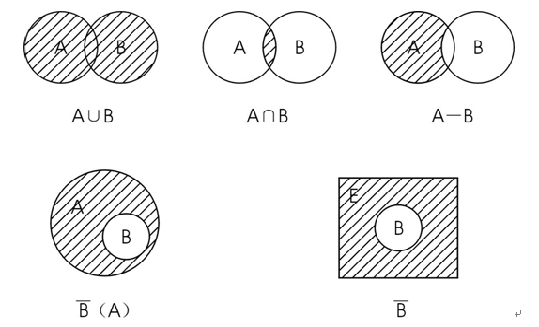（2）幂集
幂集：集合A的幂集为A的所有子集之集，记

2

A

2^A

例：
A={a,b,c}， 则

2

A

=

{

B

∣

B

⊆

A

}

=

{

Θ

,

{

a

}

,

{

b

}

,

{

c

}

,

{

a

,

b

}

,

{

a

,

c

}

,

{

b

,

c

}

,

{

a

,

b

,

c

}

}

2^A= \{ B∣B \subseteq A\}=\{ \Theta ,\{a\},\{b\},\{c\},\{a,b\},\{a,c\},\{b,c\},\{a,b,c\}\}

（3）笛卡尔积
笛卡尔积：集合的笛卡尔积

A

×

B

=

{

(

a

,

b

)

∣

a

∈

A

,

b

∈

B

}

A\times B=\{(a,b)|a∈A,b∈B\}

，其中(a,b)称为有序对注意：有序对有先后关系，所以一般

A

×

B

≠

B

×

A

A\times B \neq B\times A

例：
设 A={1,2 }，B = {a,b,c}, 则

A

×

B

=

{

(

1

,

a

)

,

(

1

,

b

)

,

(

1

,

c

)

,

(

2

,

a

)

,

(

2

,

b

)

,

(

2

,

c

)

}

A\times B=\{ (1, a), (1, b), (1, c), (2, a), (2, b), (2, c) \}

（4）关系
关系R：

设

有

集

合

A

,

B

，

a

∈

A

，

b

∈

B

设有集合A,B，a∈A，b∈B

由集合A到集合B的关系，是AxB的任何子集。(a,b)∈R，写作aRb。本质上是有序对的集合，看作A到B的一个映射若A=B，称A上的关系；否则称A到B的关系  关系的k次积

R

k

R^k

：

设

有

集

合

A

，

a

1

,

a

2

∈

A

设有集合A，a_1,a_2∈A

递归定义：

a

1

R

1

a

2

a_1R^1a_2

<=>

a

1

R

a

2

a_1R a_2

a

1

R

i

a

2

a_1R^ia_2

<=>

存

在

b

∈

A

,

使

a

1

R

b

且

b

R

i

−

1

a

2

,

(

i

=

2

,

3..

k

）

存在b \in A,使a_1Rb且bR^{i-1}a_2, (i=2,3..k）

看作在A上映射了k-1次  关系的传递闭包

R

+

R^+

：

设

有

集

合

A

，

a

1

,

a

2

∈

A

设有集合A，a_1,a_2∈A

a

1

R

+

a

2

a_1R^+a_2

<=>

a

1

R

i

a

2

（

i

=

1

,

2

,

3..

）

a_1R^ia_2 （i=1,2,3..）

即

R

+

=

∪

i

≥

1

R

i

R^+=\cup_{i≥1}R^i

看作在A上映射了任意多次（不含0次）  关系的自反传递闭包

R

∗

R^*

：

设

有

集

合

A

，

a

1

,

a

2

∈

A

设有集合A，a_1,a_2∈A

规定

R

0

R^0

：

a

1

R

0

a

2

<

=

>

a

1

=

a

2

a_1R^0a_2 <=> a_1=a_2

，则

R

∗

=

R

0

∪

R

+

R^*=R^0\cup R^+

只比R+多一些形如(a,a)的，看作看作在A上映射了任意多次，（含0次）
二、字母表，字符串和语言
（1）字母表
字母表(Σ)：字母符号的有限非空集。（这里的字母符号不是指狭义的英文字符，可以是数字，也可以加号下划线之类的任何符号）
（2）字符串
字符串：在Σ上定义的符号串称字符串，定义：
ε为空串，它不含任何Σ上的符号若X为Σ上符号串，a∈Σ，则Xa是Σ上符号串Y为Σ上符号串 <=> Y由1、2推出 空串（ε）： 特殊字符串，由0个符号组成
不是空白符号( 空格也是一个符号 )任何Σ中都有ε空串和其他串拼接时失去作用：Xε=εX=X 字符串术语：
字符串的连接：若X.Y为Σ上符号串，则XY也是Σ上符号串字符串的逆转

X

R

X^R

：将字符串中各符号逆序写出，若X为单个符号串或ε，则

X

R

=

X

X^R=X

前缀：删去原字符串尾部的零个或多于零个符号后缀：删去原字符串的头部的零个或多于零个符号
(真)前/后缀：X为XY前缀；若

X

≠

X

Y

X\neq XY

，X为真前缀（X可为ε） 子串：从原字符串中删去一个前缀和一个后缀子序列：从原字符串中删去零个或多于零个符号(这些符号不要求连续）字符串长度

∣

X

∣

|X|

：字符串中字符个数字符串的幂

a

n

a^n

: 符号/字符串a连续出现n次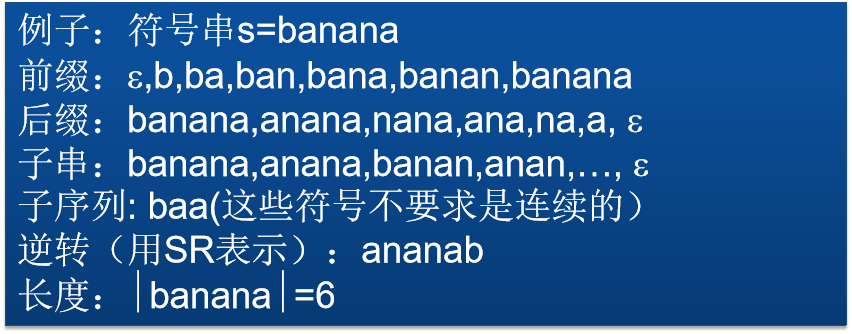（3）语言
语言(L)：对于给定字母表Σ，Σ上字符串的一个集合，称为Σ上的语言前缀性质：L为某Σ上的一个语言，若L中任意字符串都不是另一个字符串的前缀，则L有~语言的运算：
集合基本运算：交并差补（语言是特殊的集合）  语言的连接：

L

1

L_1

和

L

2

L_2

的连接

L

1

L

2

L_1L_2

由下式定义：

L

1

L

2

=

{

x

y

∣

x

∈

L

1

,

y

∈

L

2

}

L_1L_2=\{xy∣x∈L_1,y∈L_2\}

（排列组合）  语言的闭包

L

∗

L^*

： 递归定义：

L

0

=

{

ε

}

L^0=\{ε\}

对于n≥1,

L

n

=

L

L

n

−

1

L^n =LL^{n-1}

;

L

∗

=

∪

L

n

L^*=∪L^n

直观含义：以任意次序连接L中任意多个字符串，所组成的集合）  语言的正闭包

L

+

L^+

：

L

+

=

∪

L

n

(

n

>

=

1

)

，

故

L

∗

=

L

0

∪

L

+

L^+=∪L^n (n>=1)，故L^*=L^0∪L^+

注意：字母表Σ本身也是Σ上的语言，有Σ*和Σ+类似定义  
展开全文• 形式语言自动机课程笔记 学到编译原理的时候用到了文法相关概念，复习自动机正好把以前的笔记整理一下也贴上来 一、问题的提出 语言是给定字母表上字符串的一个集合 任何有意义的语言，都是符合某些规则要求的...
形式语言自动机课程笔记学到编译原理的时候用到了文法相关概念，复习自动机正好把以前的笔记整理一下也贴上来

文章目录
一、问题的提出二、形式文法和形式语言（1）形式文法（2）形式语言
三、文法等价四、文法的乔姆斯基分类五、左/右线性文法六、语法分析树和文法二义性（1）定义（2）分析（3）文法二义性

一、问题的提出
语言是给定字母表上字符串的一个集合任何有意义的语言，都是符合某些规则要求的字符串的集合我们把这种有意义的语言称作文法
文法可以用自然语言描述，如英语语法文法也可以用严格的规则形式描述，比如C语言文法，这种文法称为形式文法
二、形式文法和形式语言
（1）形式文法
文法：用有限个规则描述无穷多字符串的集合  形式文法（G）：一个文法G是一个四元组 G = ( V, T, P, S )，其中：
V：是变元的有限集。T：是终结符的有限集。P：是产生式的有限集，其中每个产生式都是α→β的形式
其中

α

∈

(

V

∪

T

)

+

α∈(V∪T)^+

，且其中至少有一个V中的符号；

β

∈

(

V

∪

T

)

∗

β∈(V∪T)^*

。 S：称为文法G的开始符号，决定这组规则最终要定义什么，S∈V。 示例：

G

1

=

(

{

A

,

B

}

,

{

0

,

1

}

,

{

A

→

0

B

,

B

→

1

B

,

B

→

0

}

,

A

)

G_1 = (\{A, B\}, \{0,1\},\{A→0B, B→1B, B→0\}, A)

G

2

=

(

{

A

,

B

,

C

}

,

{

a

,

b

}

,

{

A

→

a

B

C

,

B

→

b

,

C

→

C

C

,

C

→

ε

}

,

A

)

G2 =( \{A,B,C\},\{a,b\},\{A→aBC, B→b, C→CC, C→ε\}, A)

文法表示方式的约定：
符号表示变元大写字母ABCD…除非特殊说明，否者S表示开始符号终结符号小写字母abcde/数字123…终结符串小写字母uvwxyz变元和终结符混合串希腊字母αβγ…
若有若干产生式左部一致，记为

α

→

β

1

∣

β

2

∣

.

.

∣

β

n

α\to β_1|β_2|..|β_n

上述约定下，写一个文法只需写出产生式集合 示例：

G

=

(

{

S

}

,

{

a

,

b

}

,

{

S

→

a

S

b

,

S

→

a

b

}

,

S

)

G=(\{S\},\{a,b\},\{S→aSb,S→ab\},S)

可以表示为：S→aSb|ab  给出文法G，它的产生式是：
S→aB∣bAA→a∣bAA∣aSB→b∣aBB∣bS 则Ｌ(G)是由相等个数a和b组成的（次序不限）所有串的集合 (可以用归纳法证明)
字符串/文法推倒与规约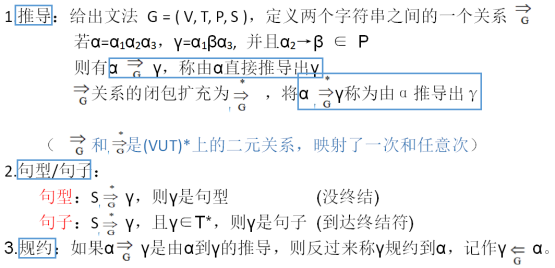最左（右）推倒：对于推导α→β，如果每一步都是对α中的最左非终结符进行替换的，则我们称这种推导为最左推导，如果每一步都是对α中的最右非终结符进行替换的，则我们称这种替换为最右推导，例如：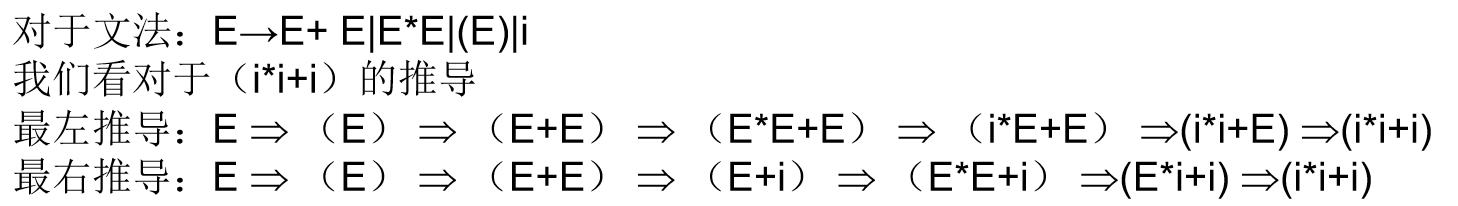（2）形式语言
形式语言：给出文法 G=(V,T,P,S)，它所产生的语言记作L(G)，由下述集合定义：

L

(

G

)

=

{

ｗ

∣

S

⟹

∗

ｗ

，

并

且

ｗ

∈

Ｔ

∗

}

L(G)=\{ｗ|S\stackrel{*}{\Longrightarrow} ｗ，并且ｗ∈Ｔ^* \}

L(G)是由G中开始符号S推导出来的全体终结符号串所构成的集合，也就是所有句子的集合。
三、文法等价
文法等价：对于两个不同的文法

G

1

＝

(

V

1

,

T

1

,

P

1

,

S

1

）

，

G

2

＝

(

V

2

,

T

2

,

P

2

,

S

2

)

，

G_1＝(V_1,T_1,P_1,S_1），G_2＝(V_2,T_2,P_2,S_2)，

如果

Ｌ

(

G

1

)

＝

Ｌ

(

G

2

)

Ｌ(G_1)＝Ｌ(G_2)

，则称文法

G

1

G_1

与

G

2

G_2

等价。在两个四元组的各对应部分中，只要有一点点不同，就应当看作是不同的文法，在这个意义下，任何一个语言都可以有无穷多个文法（都等价）产生它。
四、文法的乔姆斯基分类
对于文法　G＝（V，T，P，S）按以下方法分为四类：
0型文法，或短语结构文法（PSG）：
产生式不加另外的限制。产生短语结构语言（PSL） 1型文法，或上下文有关文法（CSG）：
产生式α→β都满足 |α|≤∣β|产生上下文有关语言（CSL） 2型文法，或上下文无关文法（CFG）：
Ｐ中每个产生式都具有如下形式：A→β，β∈(V∪T)*，A∈V产生上下文无关语言（CFL） 3型文法，或正则文法（RG）：
Ｐ中每个产生式都具有如下形式：A→a或A→aB，a∈T∪{ε}，AB∈V产生正则语言（RL）
五、左/右线性文法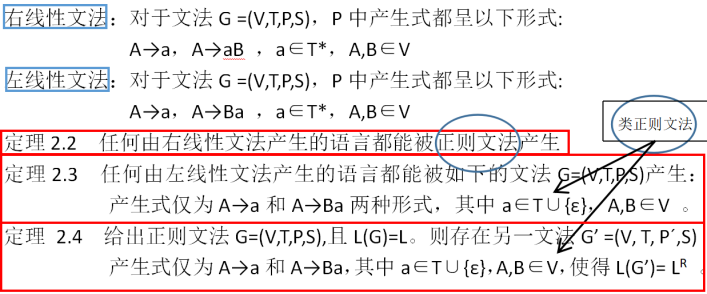六、语法分析树和文法二义性
（1）定义
分析树是推导的图形表示  设G=（V，T，S，P），G的一棵语法分析树满足如下条件：
每一结点有一标记，此标记是V∪T∪{ε}中的符号。根的标记是S。如果一个结点是内部结点（非叶节点），且标记A，那么A必在V中。如果编号为n的结点有标记A，结点

n

1

，

n

2

，

…

，

n

k

n_1，n_2，…，n_k

是点n的从左到右的儿子，并分别有标记

X

1

，

X

2

，

…

，

X

k

X_1，X_2，…，X_k

，则

A

→

X

1

X

2

…

X

k

A→X_1X_2…X_k

必须是P中的产生式。若结点n有标记ε，那么结点n是叶子，且是它父亲唯一的儿子。  边缘：一颗语法分析树，将其叶子上的标记从左到右收集组成的字符串称为该语法分析树的边缘
示例：
文法： E→E+E | E*E | (E) | i，句子i*i+i的语法分析树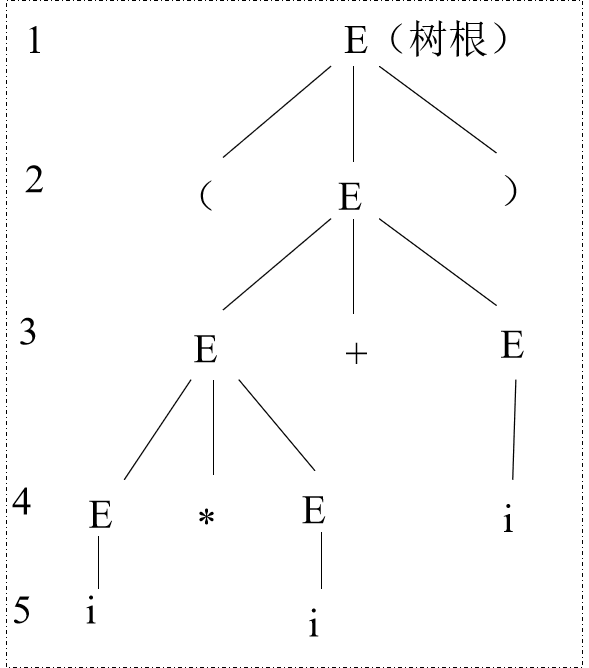（2）分析
画语法树的两种方法：
自顶向下：根据推导序列，对每步推导画相应分枝自底向上：根据归约序列，对每步归约画相应分枝 有关分析树要注意的几点：
一个句型推导或分析用一棵树结构图示出来，它反应了一个句子的语法结构层次。分析树并未描述推导过程，可以从两个角度看这个问题
对于一个句子的多种推导（若文法是无二义性的），采用各种推导过程，画出的分析树是一样的。对于同一个语法分析树，它的最左（最右）推导是唯一的。 在书中，用画分析树的过程解释语法分析过程，用分析树图解语法结构
（3）文法二义性
文法二义性：
如果一个文法的某个句子存在两棵不同的分析树，那么该句子是二义性的。如果一个文法包含二义性的句子，则称这个文法是二义性的；否则，该文法是无二义性的等价定义：文法的某字符串存在两个不同的最左（最右）推导 说明：
一般来说，程序语言要求无二义性文法，对于条件语句，经常使用二义性文法（if-else之类的）描述它对于任意一个上下文无关文法，不存在一个算法，能够在有限的步骤内判定它是无二义性的；但能给出一组充分条件，满足这组充分条件的文法是无二义性的通过给产生式引入优先级，可以把部分二义性文法转化为无二义性文法，但有一些文法是不能消除二义性的，称其为固有二义性 
展开全文• 形式语言自动机课程笔记 学到编译原理的时候用到了相关概念，复习自动机正好把以前的笔记整理一下也贴上来 一、确定型有穷自动机（DFA） （1）基本定义 确定有穷自动机DFA ：是一个五元组：M=(Q,∑,δ,q0,F)M = ...
形式语言自动机课程笔记学到编译原理的时候用到了相关概念，复习自动机正好把以前的笔记整理一下也贴上来

文章目录
〇、我的理解一、确定型有穷自动机（DFA）（1）基本定义（2）扩充定义（3）DFA接受的语言
二、非确定型有穷自动机（NFA）（1）基本定义（2）扩充定义（3）NFA接受的语言
三、具有ε转移的有穷自动机（ε-NFA）（1）基本定义（2）扩充定义（3）ε-NFA接受的语言：
四、小结

〇、我的理解
说一说我对自动机的个人理解，仅供参考
自动机是描述多状态系统的一种方法。比如指针式钟表就是一种多状态系统，它有12x60x60个状态，秒针没走一格，就切换到下一个相邻的状态。 同时，自动机是表示语言的另一种形式，具体见下面的分析  自动机的组成
既然是描述的多状态系统，那么自然有很多状态状态之间的存在转移，这种转移：
是有规则的：比如时钟只能就只能在相邻时刻，从过去向将来进行切换。比如从12:00:00切换到12:00:01，不能出现从从12:00:00切换到13:00:00之类的情况是输入驱动的：系统只有获得输入后发生状态转移，如果没有输入，就不会切换状态。还是以时钟为例，驱动转换发生的是系统获得输入“时间流逝1秒”（事实上某些系统也可能有自发的转移发生，这时要把输入定义为空ε）转换的三要素是 “转移前状态”、“输入”、“转移后状态”，有这三点就能清楚地描述一个转移
多状态系统一定有且只有一个初态，至少有一个终态。规定自动机未获得任何输入时的状态是初态（状态转换从初态开始）；终态是人为指定的，即使达到某个终态了，也可以继续接受输入并转换到自己、其他终态或非终态对于某些状态，无论输入什么，都不能驱动其最终转换到终态(经过任意多次状态转换都不行），这种状态称它为死状态。  对多状态系统进行抽象：
有若干状态存在若干输入状态间存在由输入驱动的转移有一个特殊的初始态有若干特殊的终结态  和语言的联系：除了之前介绍的文法外，自动机是表示语言的另一种形式。
语言说白了就是一些字符串的集合，只要设计一个"系统"，可以把某些字符串从世界上无穷多的字符串中提取出来，就可以说这个"系统"能表示语言。我们把字符串拆开为一个个字符，每个字符作为一个输入。自动机从初始态开始，受到每一个字符的输入不停进行状态转移，当字符串全部输入后，如果自动机处于某个终结状态，我们就说自动机接受了这个字符串。所有自动机接受的字符串的集合称为这个自动机描述的语言  自动机的分类：基于状态转移规则形式上的不同进行分类
一、确定型有穷自动机（DFA）
（1）基本定义
确定有穷自动机DFA ：是一个五元组：

M

=

(

Q

,

∑

,

δ

,

q

0

,

F

)

M = (Q,∑,δ,q_0,F)

，其中

Q

Q

是有穷状态集；

∑

∑

是有穷的输入字母表

δ

δ

是转移函数，它进行全映射

Q

×

∑

→

Q

Q×∑→Q

q

0

∈

Q

q_0∈Q

，是初始状态；

F

⊆

Q

F\subseteq Q

，是终结状态集。(终止状态，接受状态)  DFA的表示方法
状态转换函数状态转移矩阵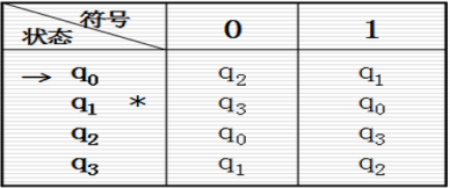状态转移图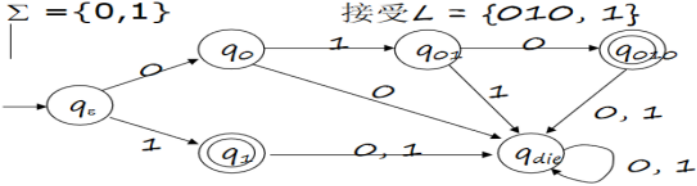1. 是一张有限方向图，结点代表状态，用圆圈表示。状态之间用箭弧连结。 2. 箭弧上的标记（字符或正规式）代表在射出结点（即箭符始结点）状态下可能出现的输入字符或字符类。 3. 初态，用双线箭头指示，表示分析的开始 4. 终态，用双圈表示，表示分析的结束 5. 一张状态转换图只包含有限个状态（即有限个结点），其中含有一个初态和若干个终态（至少一个）。 6. 一个状态转换图可用于识别（或接受）一定的字符串  注意：
DFA的转移函数是全映射，也就是说：对于n个字母表元素，如果不省略死状态，DFA每个状态一定有n个转移（出箭头）
（2）扩充定义
扩充转移函数

δ

^

\hat{\delta}

：对于DFA

M

=

(

Q

,

∑

,

δ

,

q

0

,

F

)

M =(Q,∑,δ,q_0,F)

，它的扩充转移函数 是从

Q

×

∑

∗

→

Q

Q×∑^*→Q

的映射，其具体定义如下：

δ

^

(

q

,

ε

)

=

q

\hat{\delta}(q,ε)=q

δ

^

(

q

,

w

a

)

=

δ

^

(

δ

^

(

q

,

w

)

,

a

)

其

中

q

∈

Q

，

w

∈

∑

∗

，

a

∈

∑

\hat{\delta}(q,wa)=\hat{\delta}(\hat{\delta}(q,w),a) 其中q∈Q，w∈∑^*，a∈∑

简单说就是把转移函数的第二个变元扩充为字符串，表示从状态q读过串后转移到状态q’，

δ

δ

是

δ

^

\hat{\delta}

的特例  一般DFA的

δ

δ

都指扩充定义
（3）DFA接受的语言
给出DFA

M

=

(

Q

,

∑

,

δ

,

q

0

,

F

)

M =(Q,∑,δ,q_0,F)

DFA接受的字符串：若

δ

(

q

0

,

x

)

=

p

，

p

∈

F

，

x

∈

∑

∗

δ(q_0,x)=p，p∈F ，x∈∑^*

（即串x使起始状态转移到终结状态集），则称字符串x被M接受DFA接受的语言：被M接受的全部字符串构成的集合，称为被有穷自动机M接受的语言，并记为L(M)={ x ∣δ(q0,x)∈F，x∈∑*}从状态转移图角度看：状态转移图上，任何一条从某一初态结点到某一终态结点的通路，其上所有弧的标记字依序连接成的字符串（忽略那些标记为ε的弧）等于α，则称α可为DFA M所识别（读出或接受），α也可能是空串ε
二、非确定型有穷自动机（NFA）
（1）基本定义
非确定有穷自动机NFA：一个五元组

M

=

(

Q

,

∑

,

δ

,

q

0

,

F

)

M=(Q,∑,δ,q_0 , F)

其中

Q

、

∑

、

q

0

、

F

Q、∑、q_0、F

与DFA含意相同，

δ

是

从

Q

×

∑

→

2

Q

（

Q

的

幂

集

）

δ是从Q×∑→2^Q（Q的幂集）

上的映射。 注意这里状态转移函数右边是集合了，应写成集合形式{…}  说明：
NFA的运行过程中某个时刻是会同时处于多个状态中，只要输入串x结束时NFA所处的多个状态中至少有一个是接受状态，就判断NFA接受x这相比DFA增加了不确定性，加强了其表达能力。可以理解成描述同一个多状态系统时，NFA比DFA形式更简便  注意：
n个字母表元素，NFA每个状态不一定有n个转移（出箭头），有些没定义，δ为Ø
（2）扩充定义
扩充转移函数

δ

^

\hat{\delta}

：扩展为：

2

Q

×

∑

∗

→

2

Q

2^Q×∑^*→2^Q

的映射，具体定义如下

δ

^

(

q

1

,

q

2..

,

ε

)

=

q

1

,

q

2..

,

\hat{\delta}({q1,q2..},ε)={q1,q2..},

δ

^

(

q

1

,

q

2..

,

w

a

)

=

p

∣

p

∈

δ

(

r

,

a

)

，

r

∈

(

q

1

,

q

2..

.

.

,

w

)

\hat{\delta}({q1,q2..},wa)={p |p∈δ(r,a)，r∈ ({q1,q2..}..,w)}

可以看到这里变成了从集合到集合的映射，输入也从一个字符变成了字母表闭包（字符或字符串）  这样理解：假设初始状态集是

Q

o

r

g

Q_{org}

，从第一个元素

q

o

r

g

1

q_{org1}

开始读串x，读过第一个符号转移到若干状态，构成一个状态集

Q

11

Q_{11}

，再对状态集

Q

11

Q_{11}

中的n个状态，分别读取下一个符号，得到的n个状态并起来得到

Q

12

Q_{12}

。再对其中每一个状态读取下一个符号直到读完得到

Q

1

n

Q_{1n}

，则

δ

^

(

q

1

,

x

)

=

Q

1

n

\hat{\delta}(q1,x)=Q_{1n}

，依次处理状态集

Q

o

r

g

Q_{org}

中所有初始状态，得到

Q

1

n

、

Q

2

n

、

Q

3

n

.

.

.

Q_{1n}、Q_{2n}、Q_{3n}...

，全部取并即可  一般NFA的

δ

δ

都指扩充定义
（3）NFA接受的语言
给出NFA

M

=

(

Q

,

∑

,

δ

,

q

0

,

F

)

，

x

是

∑

上

的

一

个

字

符

串

M=(Q,∑,δ,q_0 , F)，x是∑上的一个字符串

NFA接受的字符串：若

δ

(

q

0

,

x

)

∩

F

≠

ϕ

，

（

x

∈

∑

∗

）

δ(q_0,x)∩F\neq \phi，（x∈∑^*）

，则称字符串x被M接受被NFA接受的语言L(M）：被NFA M接受的全体字符串称为M接受的语言
三、具有ε转移的有穷自动机（ε-NFA）
（1）基本定义
具有ε转移的有穷自动机（ε-NFA）：是一个五元组

M

=

(

Q

,

∑

,

δ

,

q

0

,

F

)

M=(Q,∑,δ,q_0 , F)

其中Q,∑,q0,F的含义与一般的DFA或 NFA相同δ是映射

Q

×

(

∑

∪

{

ε

}

)

→

2

Q

Q×(∑∪\{ε\})→2^Q

。  说明：
和普通NFA相比，可能出现自发转移ε，即没有输入也有可能发生转移动作这相比普通NFA增加了不确定性，加强了其表达能力。  注意：
n个字母表元素，NFA每个状态不一定有n个转移（出箭头），有些没定义，δ为Ø
（2）扩充定义
ε-CLOSURE(q)：有ε-NFA，对于状态q，它的ε-CLOSURE(q)是：
形式化定义：
q在ε-CLOSURE(q)中；若p在ε-CLOSURE(q)中，则δ(p,ε)也都在ε-CLOSURE(q)中；重复2，直到ε-CLOSURE(q)中状态不再增加为止。 进一步地，对于状态集P的ε-CLOSURE( P)，我们规定：ε-CLOSURE( P) = ∪ε-CLOSURE(q)，也就是所有元素的ε-CLOSURE并起来从转移图上看，就是从状态q出发，沿着标有ε的有向边所能达到的一切状态所构成的集合（包括状态q本身）  扩充转移函数

δ

^

\hat{\delta}

：扩展为映射

2

Q

×

∑

∗

→

2

Q

2^Q×∑^*→2^Q

，定义如下：

δ

^

\hat{\delta}

(q,ε)=ε-CLOSURE(q),

δ

^

\hat{\delta}

(q,wa)=ε-CLOSURE( P), P =

∪

δ

^

\cup \hat{\delta}

(r,a)，r∈

δ

^

\hat{\delta}

(q,w)（q∈Q, a∈∑, w∈∑*）
这样理解：假设初始状态集是

Q

o

r

g

Q_{org}

，从第一个元素

q

o

r

g

1

q_{org1}

开始读串x，先扩展

q

o

r

g

1

q_{org1}

（读ε），然后对结果集合中每一个读第一个字符计算转移状态集，然后全部并（得

P

1

P_1

）,对所得集合中每个做ε扩展，再合并（至此得到

q

o

r

g

1

q_{org1}

读一个字符的结果），对结果集合中每一个读第二个字符计算转移状态集，然后求并/每个扩展/求并。重复“每个元素读入一个/求并/扩展/求并”，直到读完对于ε-NFA，

δ

^

\hat{\delta}

和

δ

\delta

必须要区分
（3）ε-NFA接受的语言：
给出ε-NFA

M

=

(

Q

,

∑

,

δ

,

q

0

,

F

)

M=(Q,∑,δ,q_0 , F)

，它接受的语言定义为：
被ε-NFA接受的语言L(M） ：

L

(

M

)

=

{

w

∣

(

q

0

,

w

)

∩

F

≠

ϕ

}

L(M)=\{w | (q0,w)∩F\neq \phi\}

四、小结
DFA、NFA、ε-NFA表达能力依次提高，因为引入的不确定性越来越高。三者的接受能力没有变化，换句话说这三者是等价的
展开全文• 形式语言自动机理论教学参考书(蒋宗礼 ,清华大学出版社)高清pdf版，另外我还把搜集的前四章的详细答案一并打包。形式语言有这些就足够了。
• 非常好的自动机理论教程，中文版，是学习形式语言 编译原理的必备教材
• 上述从有限自动机构造正则表达式的中间状态消去法的实例：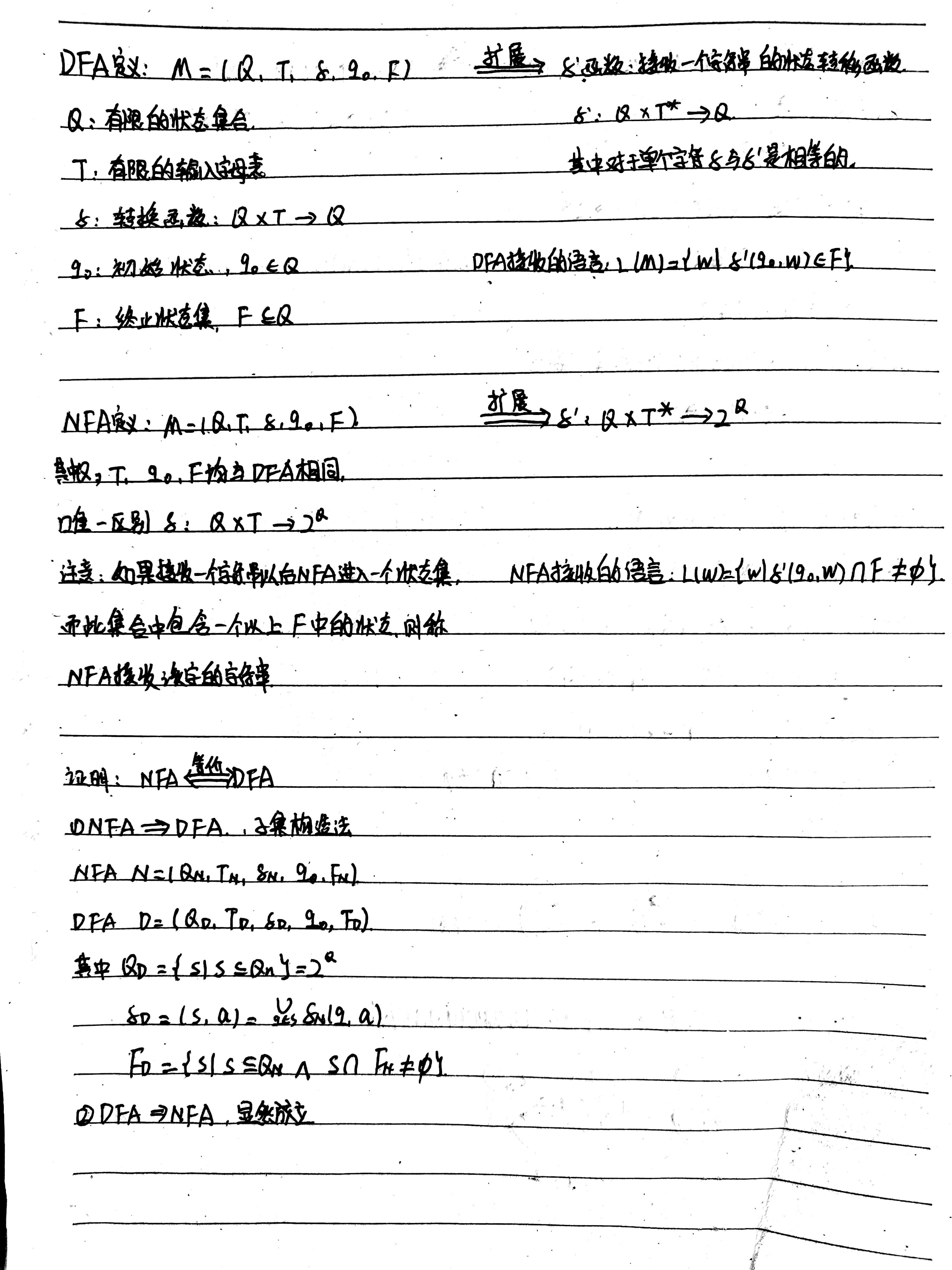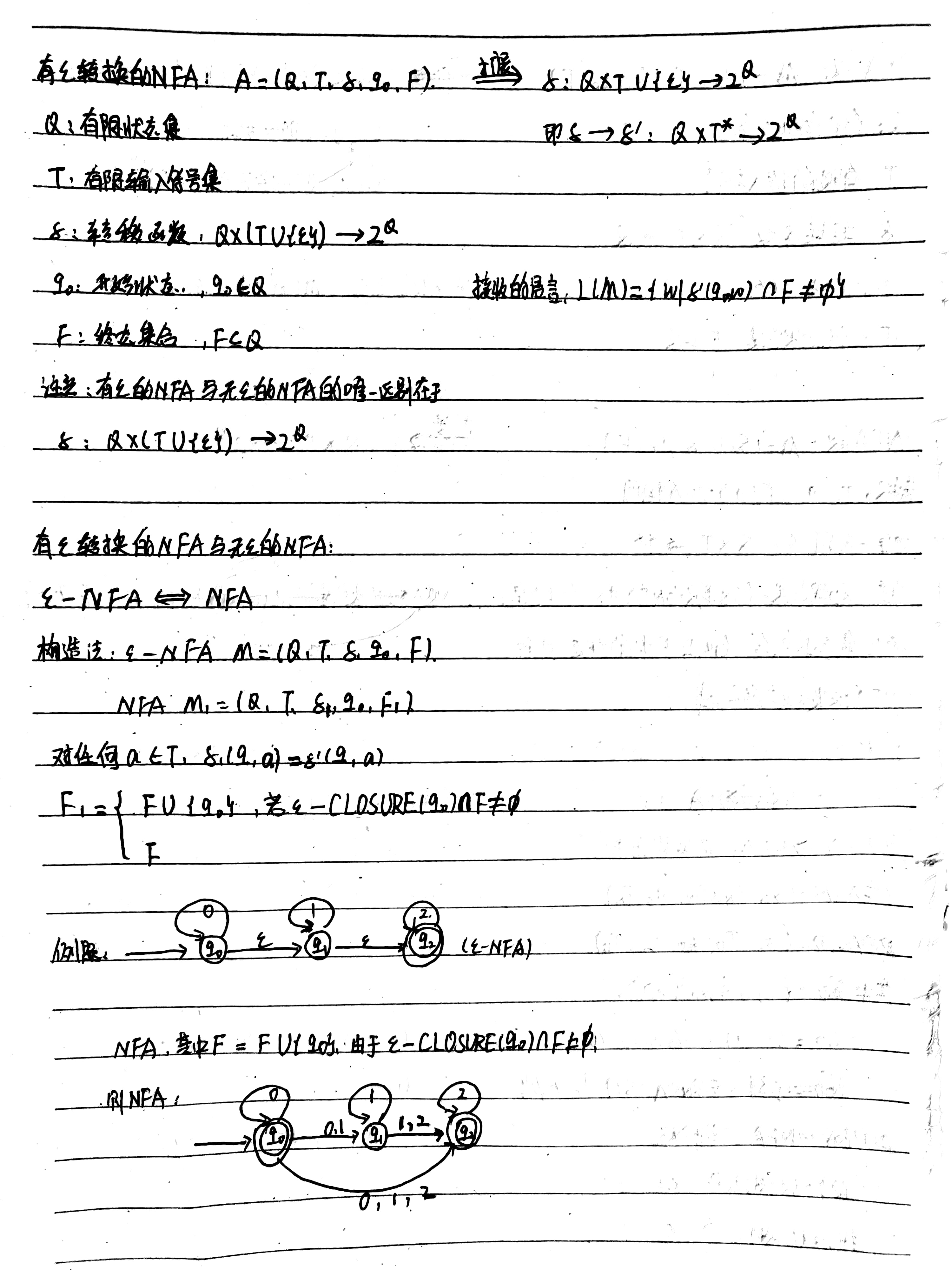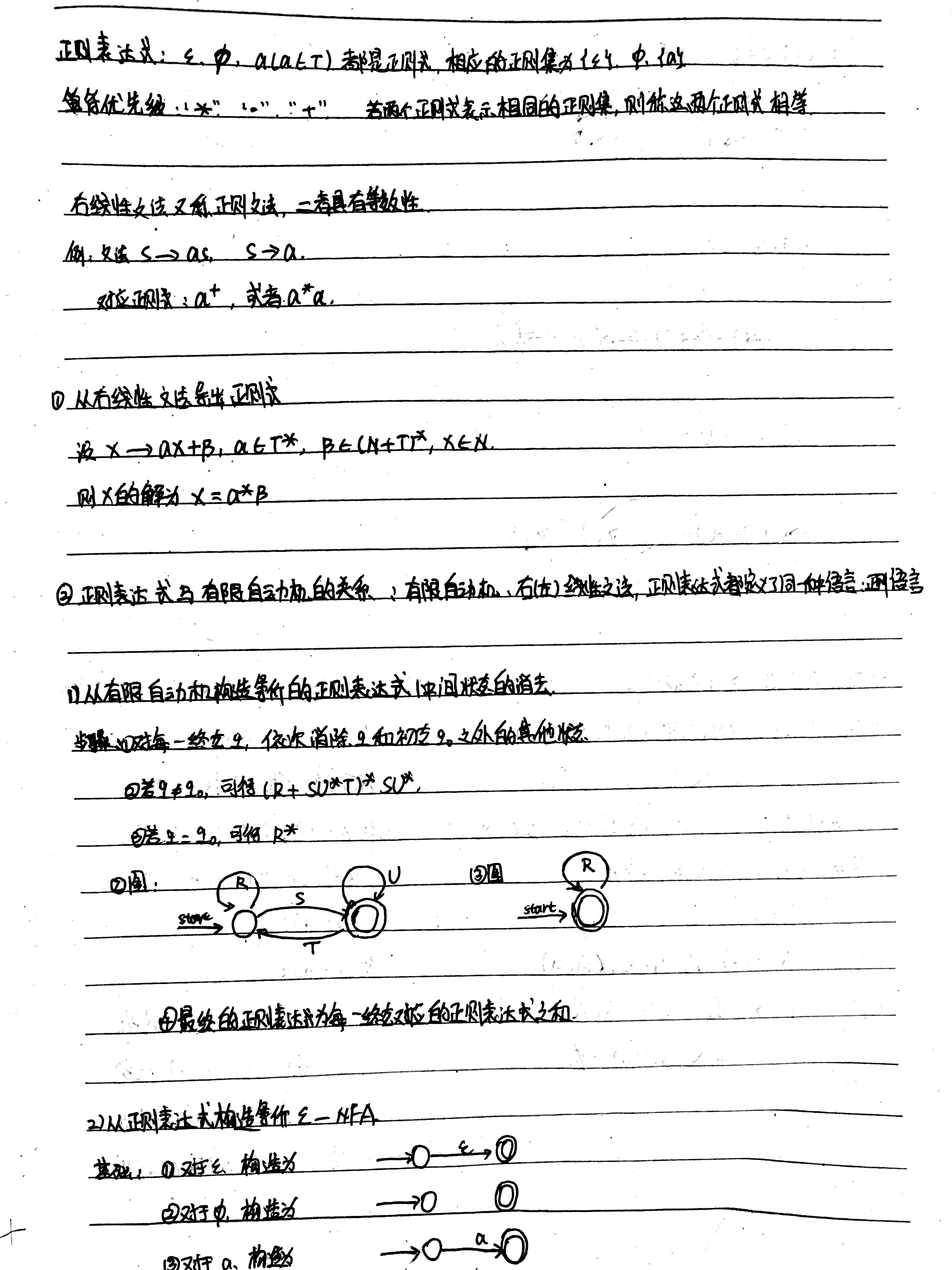上述从有限自动机构造正则表达式的中间状态消去法的实例：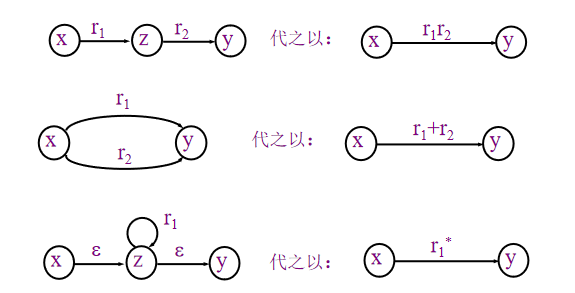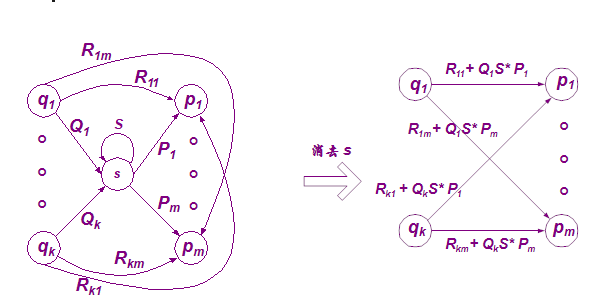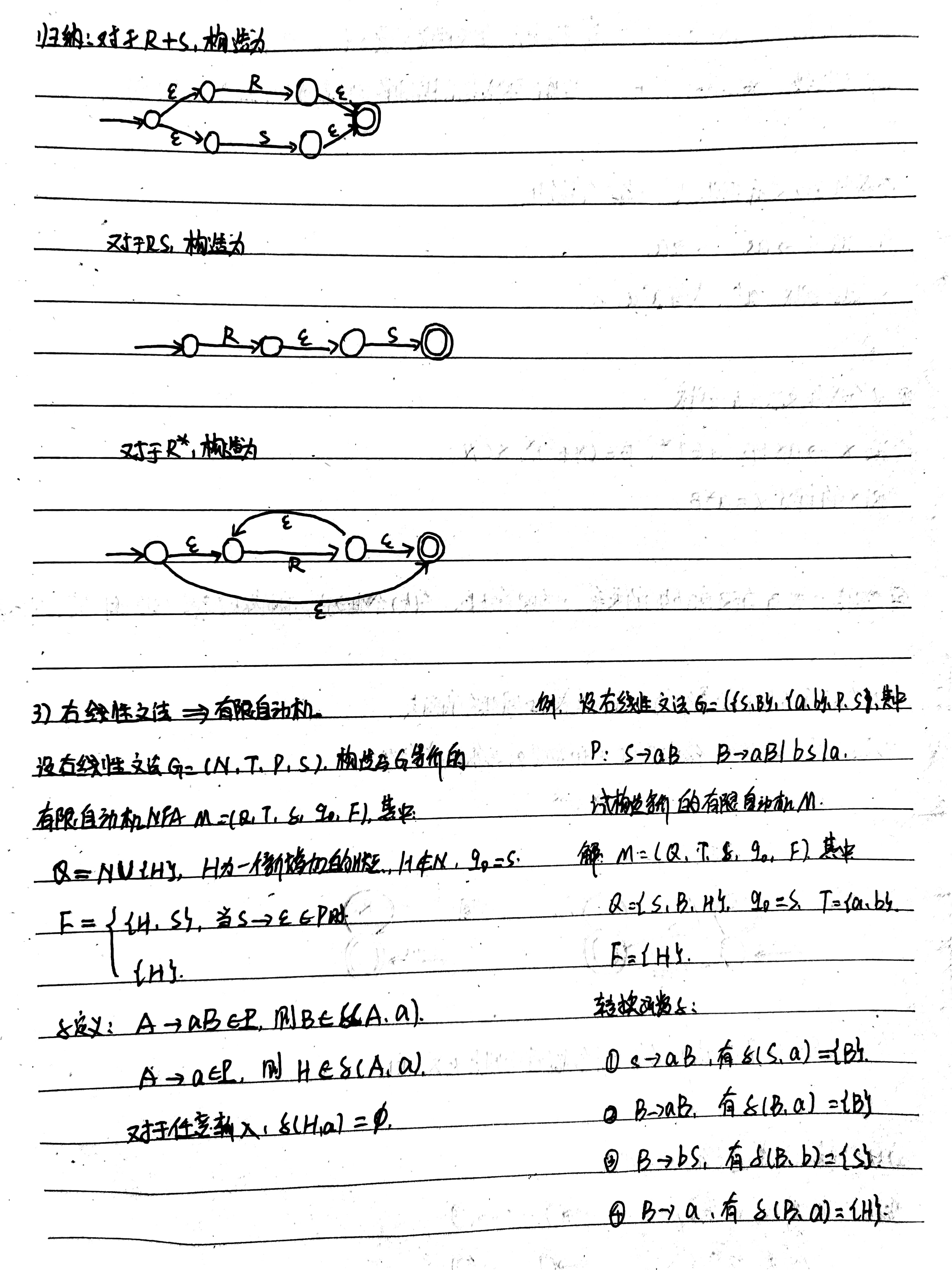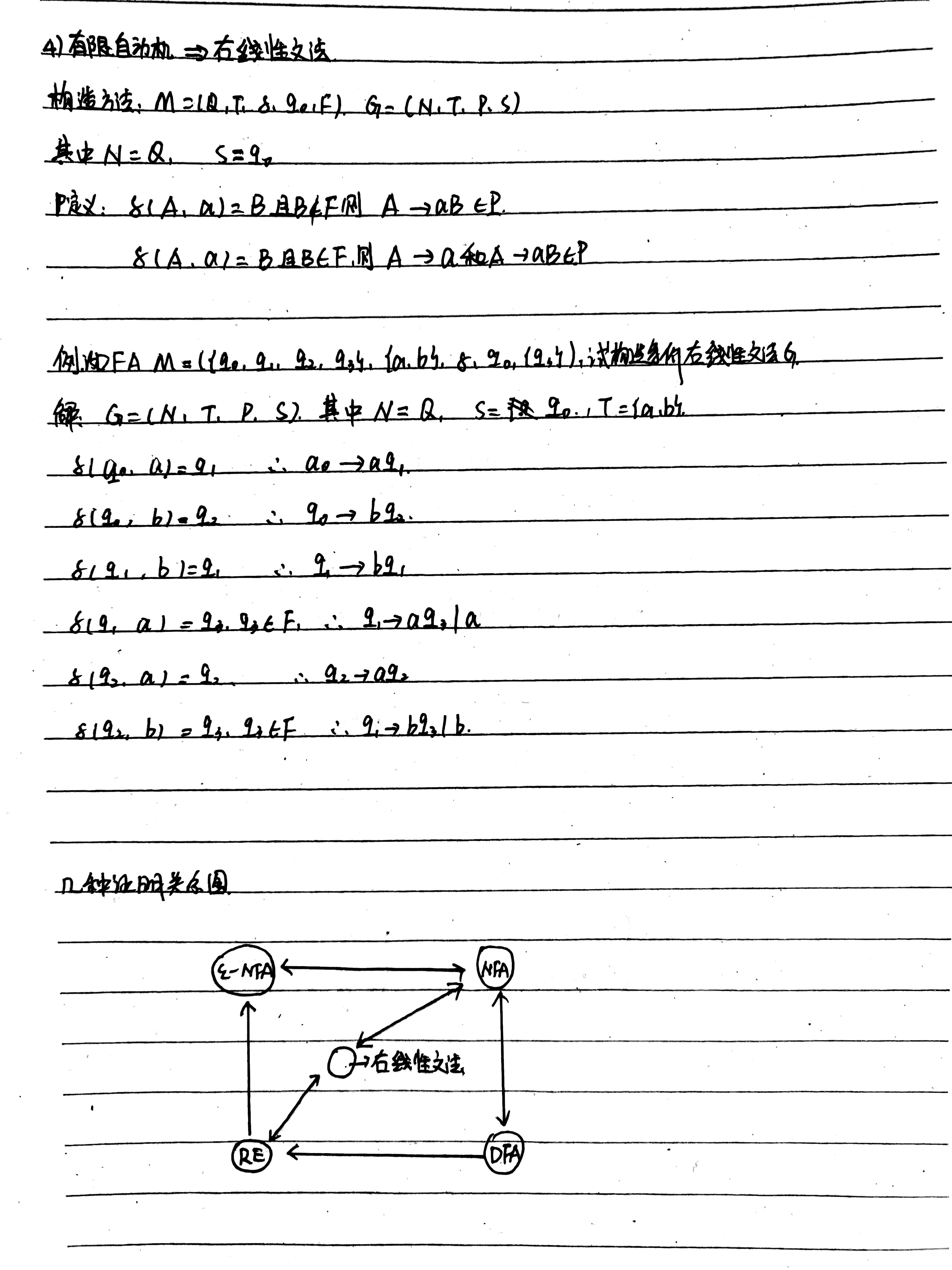`
展开全文• 1.形式语言：是用来精确地描述语言（包括人工语言和自然语言）及其结构的手段。形式语言学 也称代数语言学。 2.自动机：识别器是有穷地表示无穷语言的另一种方法。每一个语言的句子都能被一定的识别器所接受。 *有限...
• 不要浪费时间了，你要的我这里全打包好了，内含课后题答案详解+精炼讲义，是非常实用的素材，因为学习的时候感觉自己因为资料不足而很耗费精力，所以发上来帮助学弟学妹摆脱抽象的烦恼。
• 形式语言自动机的完整课件，有助于理解语言和自动机的关系。
• 蒋宗礼形式语言自动机课后答案，答案是前四章，网上至今没有流出五章之后的答案，希望有能人志士可以补充后面的答案
• 形式语言 自动机 第二版 ppt课件 欢迎大家下载
• 形式语言自动机 答案 形式语言自动机 答案 形式语言自动机 答案 形式语言自动机 答案
• 形式语言自动机课件形式语言自动机课件形式语言自动机课件形式语言自动机课件
• 形式语言自动机理论 形式语言自动机理论 形式语言自动机理论 形式语言自动机理论
• 本书采用通俗的语言和形象化的方法来表达概念和定理，逻辑严谨、思维缜密，可作为高等院校计算机及相关专业“形式语言自动机”课程的教材。  作者简介编辑 陈有祺，南开大学信息技术科学学院教授，多年来一直...
• 本人的第一手资料，绝对有用。有关课本的配套课件，绝对完整。...